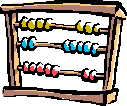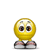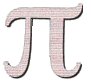MATH HUMOUR
MATH HUMOUR HOME

MAIN
HOME
TESTS
TUTORIALS
SAMPLE PROBLEMS
COMMON MISTAKES
STUDY TIPS
GLOSSARY
APPLICATIONS
MATH HUMOUR

# Math Humour!This page includes a few mathematically humourous jokes and sayings that any mathematician can appreciate! The jokes contain something essential about mathematics and the mathematical way of thinking.

Enjoy!Q: What did zero say to eight?
A: Nice belt!!

Q: What do you call a mathematician's bird that won't eat?
A: A poly "no meal"

Q: How does one insult a mathematician?
A: You say: "Your brain is smaller than any ε > 0"Q: Why do you rarely find mathematicians spending time at the beach?
A: Because they have sine and cosine to get a tan and don't need the sun!

Life is complex: it has both real and imaginary components.

Q: How do mathematicians induce good behavior in their children?
A: 'If I've told you n times, I've told you n+1 times...'

Theorem: Every positive integer is interesting.
Proof: By contradiction, assume that there exists an uninteresting positive integer. Then there must be a smallest uninteresting positive integer. But that's pretty interesting! Therefore a contradiction!

Q: What is purple and commutative?
A: An abelian grape...Let epsilon be less than zero...

In some alley, a function meets up with a differential operator:
"Get out of my way - or I'll differentiate you till you're zero!"
"Try it - I'm ex..."

Top ten excuses for not doing homework:I accidentally divided by zero and my paper burst into flames.
It was Isaac Newton's birthday.
I could only get arbitrarily close to my textbook. I couldn't actually reach it.
I have the proof, but there isn't room to write it in this margin.
I was watching the World Series and got tied up trying to prove that it converged.
I have a solar powered calculator and it was cloudy.
I locked the paper in my trunk but a four-dimensional dog got in and ate it.
I couldn't figure out whether I am the square root of negative one or i is the square root of negative one.
I took time out to snack on a doughnut and a cup of coffee. I spent the rest of the night trying to figure which one to dunk.
I could have sworn I put the homework inside a Klein bottle, but this morning I couldn't find it.

Salary Theorem: The less you know, the more you make.
Proof:
Fact #1: Knowledge is Power
Fact #2: Time is Money
We know that: Power = Work / Time
And since Knowledge = Power and Time = Money
It is therefore true that Knowledge = Work / Money
Solving for Money, we get:
Money = Work / Knowledge
Thus, as Knowledge approaches zero, Money approaches infinity, regardless of the amount of Work done

Q: What is the world's longest song?A: "Aleph-nought Bottles of Beer on the Wall."

Q: How many mathematicians does it take to screw in a light bulb?
A1: None. It's left to the reader as an exercise.
A2: None. A mathematician can't screw in a light bulb, but he can easily prove the work can be done.
A3: One. He gives it to four programmers, thereby reducing the problem to the already solved
A4: The answer is intuitively obvious

Q: Why do they never serve beer at a math party?
A: Because you can't drink and derive...

A SLICE OF PI
******************
3.14159265358979
1640628620899
23172535940
881097566
5432664
09171
036
5

Top ln(e^10) reasons why e is better than pi:10) e is easier to spell than pi.
9) pi ~= 3.14 while e ~=2.718281828459045...
8) The character for e can be found on a keyboard, but pi sure can't.
7) Everybody fights for their piece of the pie.
6) ln(pi) is a really nasty number, but ln(e) = 1.
5) e is used in calculus while pi is used in baby geometry.
4) 'e' is the most commonly picked vowel in Wheel of Fortune.
3) e stands for Euler's Number, pi doesn't stand for squat.
2) You don't need to know Greek to be able to use e.
1) You can't confuse e with a food product.

Some famous mathematician was to give a keynote speech at a conference. When he was asked for an advance summary, he said he would present a proof of Fermat's Last Theorem -- but they shouldn't announce it. Although when he arrived, he spoke on a much more prosaic topic. Afterwards the conference organizers asked why he said he'd talk about the theorem and then didn't. He replied that this was his standard practice, just in case he was killed on the way to the conference.

An engineer thinks that his equations are an approximation to reality.
A physicist thinks reality is an approximation to his equations.
A mathematician doesn't care.Did you hear about the teacher who was arrested trying to board an airplane with a compass, a protractor and a calculator?

He was charged with carrying weapons of math instruction.

If it's zero degrees outside today and it's supposed to be twice as cold tomorrow, how cold is it going to be?

A mathematician organizes a lottery in which the prize is an infinite amount of money. When the winning ticket is drawn, and the jubilant winner comes to claim his prize, the mathematician explains the mode of payment:
"1 dollar now, 1/2 dollar next week, 1/3 dollar the week after that..."There are two groups of people in the world:
Those that don't do math... and those that take care of them.

The Dictionary: What mathematics professors say, and what they mean by it:
Clearly: I don't want to write down all the "in-between" steps.
Trivial: If I have to show you how to do this, you're in the wrong class.
It can easily be shown: No more than four hours are needed to prove it.
Check for yourself: This is the boring part of the proof, so you can do it on your own time.
Hint: The hardest of several possible ways to do a proof.
Brute force: Four special cases, three counting arguments and two long inductions.
Elegant proof: Requires no previous knowledge of the subject matter and is less than ten lines long.
Similarly: At least one line of the proof of this case is the same as before.
Two line proof: I'll leave out everything but the conclusion, you can't question 'em if you can't see 'em.
Briefly: I'm running out of time, so I'll just write and talk faster.
Proceed formally: Manipulate symbols by the rules without any hint of their true meaning.
Proof omitted: Trust me. It's true.Math Love:

1. Honey, you're sweeter than 3.14
2. I'm not trying to be obtuse, but you're acute girl.
3. You and I would add up better than a Riemann sum.
4. I'll love you from here to infinity
5. You fascinate me more then the Fundamental Theorem of Calculus.
6. Are you a differential function? Because I'd like to be tangent to your curves!
7. I am equivalent to the Empty Set when you aren't with me.

|Top of Page |

COURSE HOMEPAGE
MATH 1046

FACULTY HOMEPAGES
Alex Karassev
Ted Chase